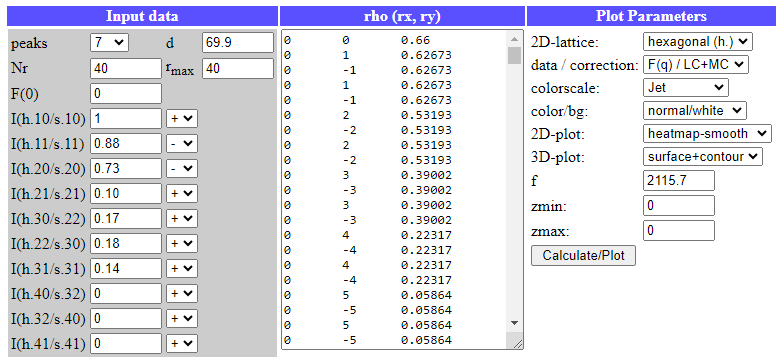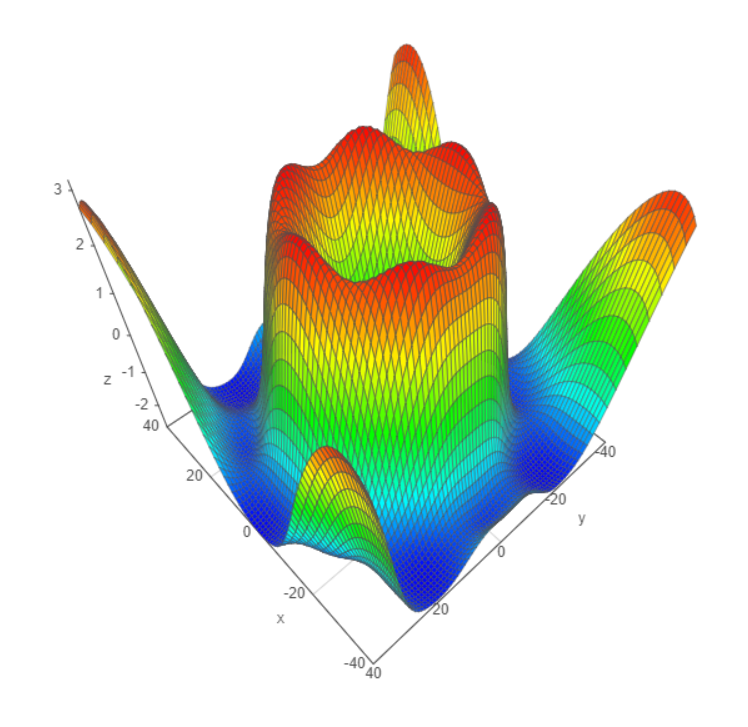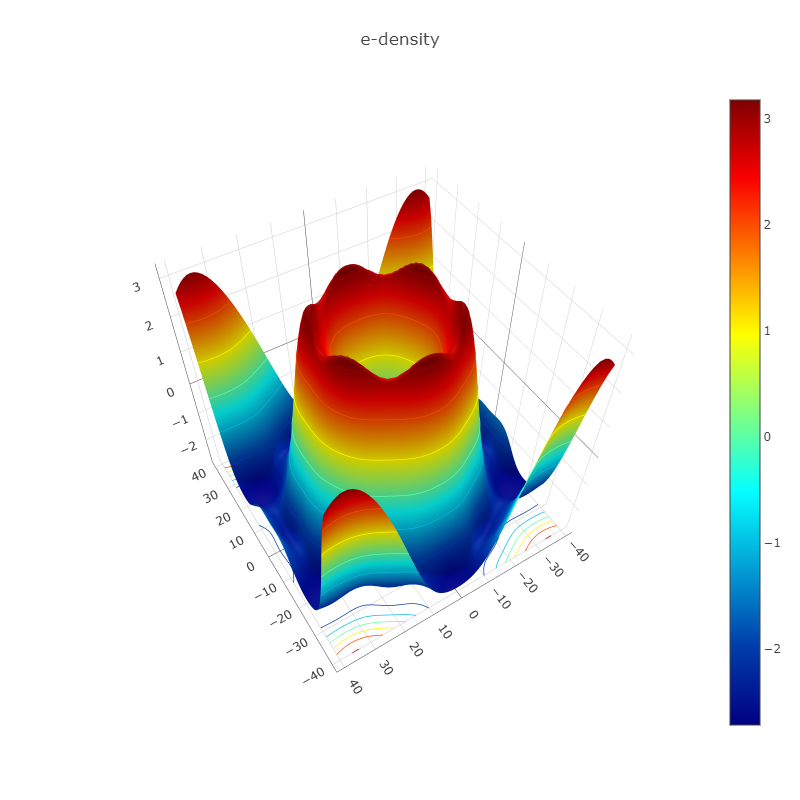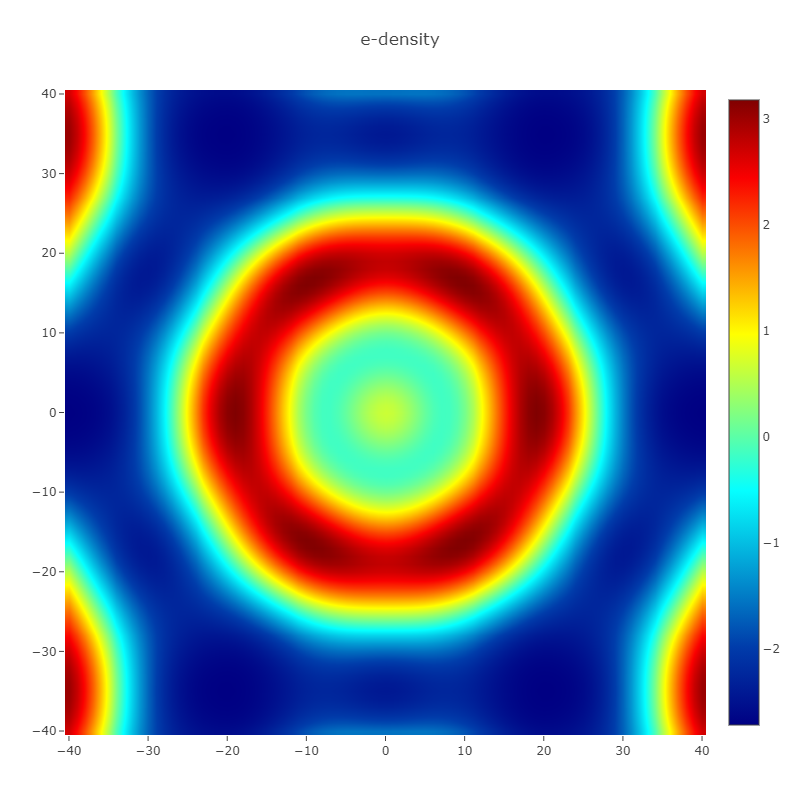## Calculation of the Electron Density of a Hexagonal Unit Cell from Diffraction Data (Example).

As an example, the electron density of a hexagonal unit cell is calculated from (X-ray) diffraction data. We take the experimental data from the paper Harper et al. 2001, Biophysical Journal, 81, 2693–2706, for fully hydrated aqueous dispersions of the phospholipid DEPE (Di-“elaidoyl”-phosphatidyl-ethanolamine) at 85°C. The first 7 diffraction peaks of the hexagonal phase are given with 1.00 / 0.88 / 0.73 / 0.10 / 0.17 / 0.18 / 0.14, which are the Lorentz- and multiplicity-corrected amplitudes with the signs +/-/-/+/+/+/+. The hexagonal lattice spacing of the lipid at 85°C is 69.9 A.

The parameters for the calculation are entered as input into the Electron density calculator for 2D-hexagonal or 2D-square lattices as shown below:The results (e-density of the hexagonal phase) are plotted in 3D and 2D:Author: M.Kriechbaum, TU-Graz (2020), e-mail: manfred.kriechbaum@tugraz.at